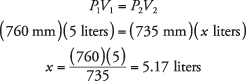## Boyle's Law

Pressure is the amount of force exerted on one unit of area. The example of an ocean diver should make the concept clearer: The greater the depth the diver reaches, the greater the pressure due to the weight of the overlying water. Pressure is not unique to liquids but can be transmitted by gases and solids, too. At the surface of the Earth, the weight of the overlying air exerts a pressure equal to that generated by a column of mercury 760 mm high. The two most common units of pressure in chemical studies are atmosphere and millimeters of mercury.

1 atm = 760 mm Hg

However, the standard international unit for pressure is the Pascal.

1 atm = 101325 Pa

The English scientist Robert Boyle performed a series of experiments involving pressure and, in 1662, arrived at a general law—that the volume of a gas varies inversely with pressure.

PV = constant

This formulation has become established as Boyle's law. Of course, the relationship is valid only if the temperature remains constant.

As an example of the use of this law, consider an elastic balloon holding 5 L of air at the normal atmospheric pressure of 760 mm Hg. If an approaching storm causes the pressure to fall to 735 mm Hg, the balloon expands. The product of the initial pressure and volume is equal to the product of the final pressure and volume while the temperature is constant.It is important that you realize that pressure and volume vary inversely; therefore, an increase in either one necessitates a proportional decrease in the other.

• Convert a pressure of 611 mm Hg to atmospheres.
• If a gas at 1.13 atm pressure occupies 732 milliliters, what pressure is needed to reduce the volume to 500 milliliters?